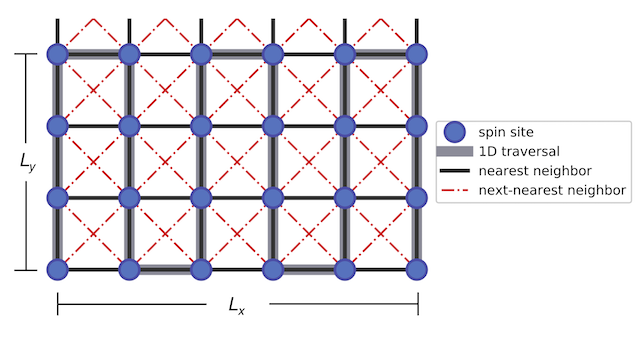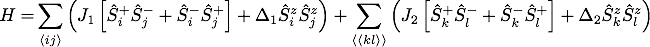DMRG.x Documentation

The following pages provide a quick overview into DMRG.x, a distributed-memory implementation of the DMRG algorithm based on the PETSc and SLEPc libraries.

Source code: https://github.com/jnvance/DMRG.x

Tutorial Resources: https://github.com/jnvance/DMRGTutorial

# Abstract

The Density Matrix Renormalization Group (DMRG) algorithm is a numerical technique used in the study of low-dimensional strongly correlated quantum systems. With this implementation, one can study two-dimensional spin systems using a one-dimensional traversal of the lattice.

In particular, the current version deals with a square-type lattice with longitudinal dimension $$L_x$$ and transverse dimension $$L_y$$ shown in the following figure. The spin sites can interact with their nearest neighbor (NN) and next-nearest neighbors (NNN), and different boundary conditions may be implemented on the two directions (such as the cylindrical boundary conditions illustrated below).The Hamiltonian for this implementation takes the form:which maps to a Heisenberg model when $$J_1 = 1/2$$ and $$J_2 = \Delta_2 = 0$$, and to the J1-J2 XY model when $$\Delta_1 = \Delta_2 = 0$$.

To reduce the computational cost, we exploit $$U(1)$$ symmetry through conservation of the total magnetization ( $$S_z$$). We also implement a matrix-free approach in the diagonalization of the superblock Hamiltonian.

# Documentation

The full documentation generated with doxygen may be viewed in the link above. To generate the documentation yourself, go to the root directory and execute

\$ make docs-default


which places the documentation in docs/default/html/index.html.

# References

This application was developed as part of the following thesis for the Master in High Performance Computing Programme:

• J. Vance. "Large-Scale Implementation of the Density Matrix Renormalization Group Algorithm." (2017). [link]

• U. Schollwöck. "The density-matrix renormalization group." Rev. Mod. Phys. 77, 259 – Published 26 April 2005 [doi]
• A E Feiguin. "The Density Matrix Renormalization Group". In: Strongly Correlated Systems. Berlin, Heidelberg: Springer Berlin Heidelberg, Apr. 2013, pp. 31–65. [link]

To learn DMRG through a simpler implementation, we suggest starting from the following Python code:

• James R. Garrison, & Ryan V. Mishmash. (2017, November 29). simple-dmrg/simple-dmrg: Simple DMRG 1.0 (Version v1.0.0). Zenodo. [link]

# Credits

Author:

This application was developed in collaboration with the Condensed Matter and Statistical Physics Section of the The Abdus Salam International Centre for Theoretical Physics, under the supervision of: